# Continuity

The definition Google finds for continuous is forming an unbroken whole; without interruption.

The concept in calculus, as transferred to functions, is similar. Roughly speaking, a continuous function is one whose graph could be drawn without having to lift (or interrupt) the pencil drawing it. nte( Consider these two graphs: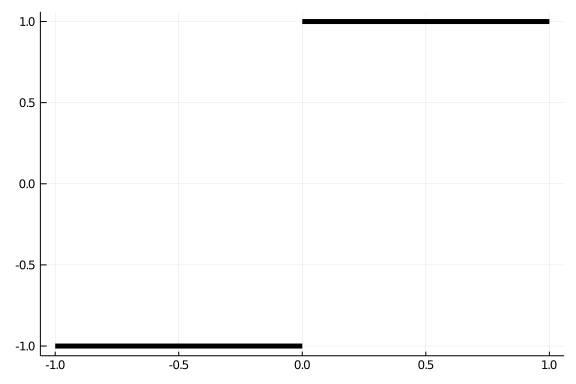and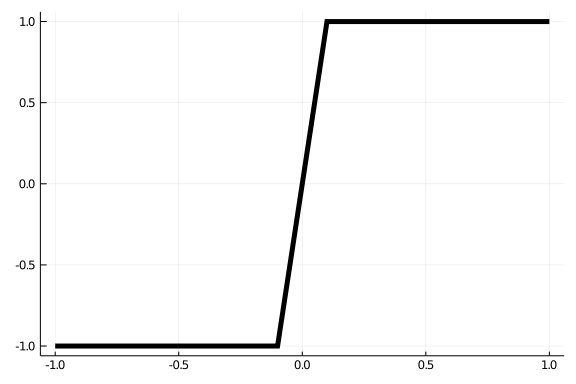Though similar at some level - they agree at nearly every value of $x$ - the first has a "jump" from $-1$ to $1$ instead of the transition in the second one. The first is not continuous at $0$ - a break is needed to draw it - where as the second is continuous.

A formal definition was a bit harder to come to. At first the concept that for any $y$ between any two values in the range for $f(x)$, the function should take the value $y$ was included. Clearly this could distinguish the two graphs above, as one takes no values in $(-1,1)$, whereas the other - the continuous one - takes on all values in that range.

However, Cauchy defined continuity by $f(x + \alpha) - f(x)$ being small whenever $\alpha$ was small. This basically rules out "jumps" and proves more useful as a tool to describe continuity.

The modern definition simply pushes the details to the definition of the limit:

A function $f(x)$ is continuous at $x=c$ if $\lim_{x \rightarrow c}f(x) = f(c)$.

This says three things

• The limit exists at $c$.

• The function is defined at $c$ ($c$ is in the domain).

• The value of the limit is the same as $f(c)$.

This speaks to continuity at a point, we can extend this to continuity over an interval $(a,b)$ by saying:

A function $f(x)$ is continuous over $(a,b)$ if at each point $c$ with $a < c < b$, $f(x)$ is continuous at $c$.

Finally, as with limits, it can be convenient to speak of right continuity and left continuity at a point, where the limit in the defintion is replaced by a right or left limit, as appropriate.

##### Examples of continuity

Most familiar functions are continuous everywhere.

• For example, a monomial function $f(x) = ax^n$ for non-negative, integer $n$ will be continuous. This is because the limit exists everywhere, the domain of $f$ is all $x$ and there are no jumps.

• Similarly, the basic trigonometric functions $\sin(x)$, $\cos(x)$ are continuous everywhere.

• So are the exponential functions $f(x) = a^x, a > 0$.

• The hyperbolic sine ($(e^x - e^{-x})/2$) and cosine ($(e^x + e^{-x})/2$) are, as $e^x$ is.

• The hyperbolic tangent is, as $\cosh(x) > 0$ for all $x$.

Some familiar functions are continuous but not everywhere.

• For example, $f(x) = \sqrt{x}$ is continuous on $(0,\infty)$ and right continuous at $0$, but it is not defined for negative $x$, so can't possibly be continuous there.

• Similarly, $f(x) = \log(x)$ is continuous on $(0,\infty)$, but it is not defined at $x=0$, so is not right continuous at $0$.

• The tangent function $\tan(x) = \sin(x)/\cos(x)$ is continuous everywhere except the points $x$ with $\cos(x) = 0$ ($\pi/2 + k\pi, k$ an integer).

• The hyperbolic co-tangent is not continuous at $x=0$ when $\sinh$ is $0$,

• The semicircle $f(x) = \sqrt{1 - x^2}$ is continuous on $(-1, 1)$. It is not continous at $-1$ and $1$, though it is right continuous at $-1$ and left continous at $1$.

##### Examples of discontinuity

There are various reasons why a function may not be continuous.

• The function $f(x) = \sin(x)/x$ has a limit at $0$ but is not defined at $0$, so is not continuous at $0$. The function can be redefined to make it continuous.

• The function $f(x) = 1/x$ is continuous everywhere except $x=0$.

• A rational function $f(x) = p(x)/q(x)$ will be continuous everywhere except where $q(x)=0$.

• The function

$~ f(x) = \begin{cases} -1 & x < 0 \\ 0 & x = 0 \\ 1 & x > 0 \end{cases} ~$

is implemented by Julia's sign function. It has a value at $0$, but no limit at $0$, so is not continuous at $0$. Furthermore, the left and right limits exist at $0$ but are not equal to $f(0)$ so the function is not left or right continuous at $0$. It is continous everywhere except at $x=0$.

• Similarly, the function defined by this graph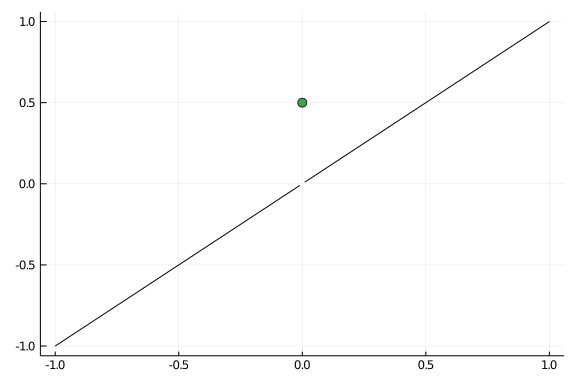is not continuous at $x=0$. It has a limit of $0$ at $0$, a function value $f(0) =1/2$, but the limit and the function value are not equal.

• The floor function, which rounds down to the nearest integer, is also not continuous at the integers, but is right continuous at the integers, as, for example, $\lim_{x \rightarrow 0+} f(x) = f(0)$. This graph emphasizes the right continuity by placing a point for the value of the function when there is a jump: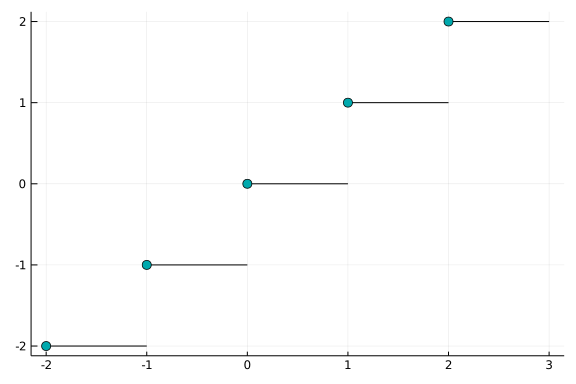• The function $f(x) = 1/x^2$ is not continuous at $x=0$: $f(x)$ is not defined at $x=0$ and $f(x)$ has no limit at $x=0$ (in the usual sense).

• On the Wikipedia page for continuity the example of Dirichlet's function is given:

$~ f(x) = \begin{cases} 0 & \text{if } x \text{ is irrational,}\\ 1 & \text{if } x \text{ is rational.} \end{cases} ~$

The limit for any $c$ is discontinuous, as any interval about $c$ will contain both rational and irrational numbers so the function will not take values in a small neighborhood around any potential $L$.

##### Example

Let a function be defined by cases:

$~ f(x) = \begin{cases} 3x^2 + c & x \geq 0,\\ 2x-3 & x < 0. \end{cases} ~$

What value of $c$ will make $f(x)$ a continuous function?

To be continuous we not that for $x < 0$ and for $x > 0$ the function is a simple polynomial, so is continous. At $x=0$ to be continuous we need a limit to exists and be equal to $f(0)$, which is $c$. A limit exists if the left and right limits are equal. This means we need to solve for $c$ to make the left and right limits equal.

using CalculusWithJulia   # load SymPy
using Plots
@vars x c
ex1 = 3x^2 + c
ex2 = 2x-3
del = limit(ex1, x=>0, dir="+") - limit(ex2, x=>0, dir="-")

\begin{equation*}c + 3\end{equation*}

We need to solve for $c$ to make del zero:

solve(del, c)

$\left[ \begin{array}{r}-3\end{array} \right]$

This gives the value of $c$.

## Rules for continuity

As we've seen, functions can be combined in several ways. How do these relate with continuity?

Suppose $f(x)$ and $g(x)$ are both continuous on $(a,b)$. Then

• The function $h(x) = \alpha f(x) + \beta g(x)$ is continuous on $(a,b)$ for any real numbers $\alpha$ and $\beta$;

• The function $h(x) = f(x) \cdot g(x)$ is continuous on $(a,b)$; and

• The function $h(x) = f(x) / g(x)$ is continuous at all points $c$ in $(a,b)$ where $g(c) \neq 0$.

• The function $h(x) = f(g(x))$ is continuous at $x=c$ if $g(x)$ is continuous at $c$ and $f(x)$ is continous at $g(c)$.

So, continuity is preserved for all of the basic operations except when dividing by $0$.

##### Examples
• Since a monomial $f(x) = ax^n$ ($n$ a non-negative integer) is continuous, by the first rule, any polynomial will be continuous.

• Since both $f(x) = e^x$ and $g(x)=\sin(x)$ are continuous everywhere, so will be $h(x) = e^x \cdot \sin(x)$.

• Since $f(x) = e^x$ is continuous everywhere and $g(x) = -x$ is continuous everywhere, the composition $h(x) = e^{-x}$ will be continuous everywhere.

• Since $f(x) = x$ is continuous everywhere, the function $h(x) = 1/x$ - a ratio of continuous functions - will be continuous everywhere except possibly at $x=0$ (where it is not continuous).

• The function $h(x) = e^{x\log(x)}$ will be continuous on $(0,\infty)$, the same domain that $g(x) = x\log(x)$ is continuous. This function (also written as $x^x$) has a right limit at $0$ (of $1$), but is not right continuous, as $h(0)$ is not defined.

## Questions

###### Question

Let $f(x) = \sin(x)$ and $g(x) = \cos(x)$. Which of these is not continuous everywhere?

$~ f+g,~ f-g,~ f\cdot g,~ f\circ g,~ f/g ~$

###### Question

Let $f(x) = \sin(x)$, $g(x) = \sqrt{x}$.

When will $f\circ g$ be continuous?

When will $g \circ f$ be continuous?

###### Question

The composition $f\circ g$ will be continuous everywhere provided:

###### Question

At which values is $f(x) = 1/\sqrt{x-2}$ not continuous?

###### Question

A value $x=c$ is a removable singularity for $f(x)$ if $f(x)$ is not continuous at $c$ but will be if $f(c)$ is redefined to be $\lim_{x \rightarrow c} f(x)$.

The function $f(x) = (x^2 - 4)/(x-2)$ has a removable singularity at $x=2$. What value would we redefine $f(2)$ to be, to make $f$ a continuous function?

###### Question

The highly oscillatory function

$~ f(x) = x^2 (\cos(1/x) - 1) ~$

has a removable singularity at $x=0$. What value would we redefine $f(0)$ to be, to make $f$ a continuous function?

###### Question

Let $f(x)$ be defined by

$~ f(x) = \begin{cases} c + \sin(2x - \pi/2) & x > 0\\ 3x - 4 & x \leq 0. \end{cases} ~$

What value of $c$ will make $f(x)$ continuous?

###### Question

Suppose $f(x)$, $g(x)$, and $h(x)$ are continuous functions on $(a,b)$. If $a < c < b$, are you sure that $lim_{x \rightarrow c} f(g(x))$ is $f(g(c))$?

###### Question

Consider the function $f(x)$ given by the following graph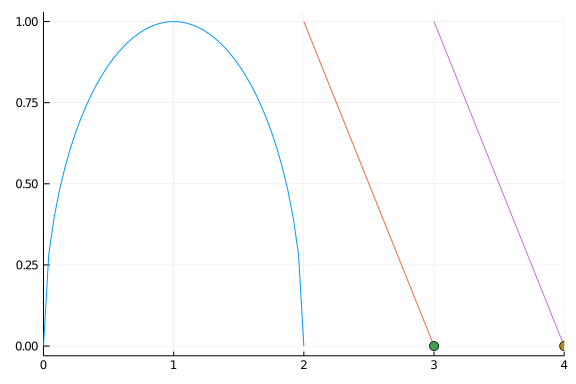The function $f(x)$ is continous at $x=1$?

The function $f(x)$ is continous at $x=2$?

The function $f(x)$ is right continous at $x=3$?

The function $f(x)$ is left continous at $x=4$?

###### Question

Let $f(x)$ and $g(x)$ be continuous functions whose graph of $[0,1]$ is given by: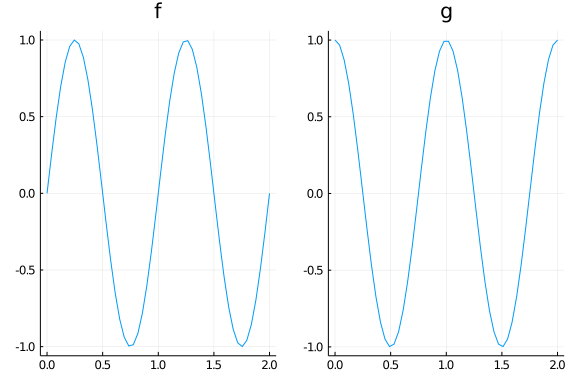What is $\lim_{x \rightarrow 0.25} f(g(x))$?

What is $\lim{x \rightarrow 0.25} g(f(x))$?

What is $\lim_{x \rightarrow 0.5} f(g(x))$?